# CorrGAN: A GAN for sampling correlation matrices (Part II)

In this blog, we do a tiny step toward the ultimate goal of sampling realistic financial correlation matrices thanks to a GAN.

For this experiment, we still restrain ourselves to 3x3 correlation matrices (as we can easily visualize them in a 3D space), but instead of learning to sample from the full 3D elliptope like in (Part I), we try to learn to sample from the 3x3 subspace of realistic financial correlation matrices.

By subspace of “realistic financial correlation matrices”, we mean the space loosely defined by millions of 3x3 empirical correlation matrices estimated on returns of the S&P500 constituents.

TL;DR It seems that the GAN do a decent job at sampling correlation matrices that could have been estimated from real returns of S&P500 constituents.

Next steps:

• Since in N-dimensions we cannot visualize easily anymore, we can try to compare the two orbifolds (original one vs. learned by GAN one) using Topological Data Analysis metrics (as in https://arxiv.org/pdf/1802.02664.pdf): Hopefully, the numbers closely match meaning the GAN might cover rather the whole subspace;

• We can monitor a few summary statistics about the GAN-generated correlation matrices, and check whether they verify the “stylized facts” (1. Large first eigenvalue, 2. Perron-Frobenius property, 3. Eigenvalues follow the Marchenko–Pastur distribution 4. Distribution of pairwise correlations is signicantly shifted to the positive, 5. Scale-free property of the corresponding MST, 6. Hierarchical structure of clusters) known in the finance literature: This will tell if they are realistic, but not that the GAN cover the whole subspace;

• Modify the neural networks so that they verify the exchangeability property, i.e. f(x1, x2, …, xn) = f(p(x1), p(x2), …, p(xn)), where p is a permutation of the n variables. Indeed, the order of the rows (and columns) of the correlation matrix is totally arbitrary (corr(A, B, C) should be equivalent to corr(B, C, A)). So, n! equivalent correlation matrices. For the 3x3, it’s not blocking as we can naively sample many equivalent matrices, but exchangeability is not an option for higher dimensions (https://arxiv.org/pdf/1703.06114.pdf).``````import pandas as pd
import numpy as np
from numpy.random import beta
from numpy.random import randn
from scipy.linalg import sqrtm
from numpy.random import seed
from random import randint

seed(42)

def sample_unif_correlmat(dimension):
d = dimension + 1

prev_corr = np.matrix(np.ones(1))
for k in range(2, d):
# sample y = r^2 from a beta distribution with alpha_1 = (k-1)/2 and alpha_2 = (d-k)/2
y = beta((k - 1) / 2, (d - k) / 2)
r = np.sqrt(y)

# sample a unit vector theta uniformly from the unit ball surface B^(k-1)
v = randn(k-1)
theta = v / np.linalg.norm(v)

# set w = r theta
w = np.dot(r, theta)

# set q = prev_corr**(1/2) w
q = np.dot(sqrtm(prev_corr), w)

next_corr = np.zeros((k, k))
next_corr[:(k-1), :(k-1)] = prev_corr
next_corr[k-1, k-1] = 1
next_corr[k-1, :(k-1)] = q
next_corr[:(k-1), k-1] = q

prev_corr = next_corr

return next_corr
``````
``````sample_unif_correlmat(3)
``````
``````array([[ 1.        ,  0.36739638,  0.1083456 ],
[ 0.36739638,  1.        , -0.05167306],
[ 0.1083456 , -0.05167306,  1.        ]])
``````

We load a total of more than 25 million 3x3 empirical correlation matrices that were estimated on returns of the S&P500 constituents. We want the GAN to learn their distribution.

``````corrs = pd.read_hdf('corr_emp_3d.h5')
``````
``````corrs.shape
``````
``````(25898310, 3)
``````
``````def sample_data(n=10000):
data = []
for i in range(n):
m = sample_unif_correlmat(3)
data.append([m[0, 1], m[0, 2], m[1, 2]])

return np.array(data)

def sample_corr(n=10000):
idx = [randint(0, len(corrs) - 1) for j in range(n)]

return corrs.loc[idx].values
``````
``````from mpl_toolkits.mplot3d import Axes3D
import matplotlib.pyplot as plt

fig = plt.figure(figsize=(10, 8))

xs = []
ys = []
zs = []
d = sample_data()
for datum in d:
xs.append(datum)
ys.append(datum)
zs.append(datum)

xc = []
yc = []
zc = []
c = sample_corr()
for corr in c:
xc.append(corr)
yc.append(corr)
zc.append(corr)

ax.scatter(xs, ys, zs, alpha=0.1)
ax.scatter(xc, yc, zc, alpha=0.2)

ax.set_xlabel('\$\\rho_{12}\$')
ax.set_ylabel('\$\\rho_{13}\$')
ax.set_zlabel('\$\\rho_{23}\$')

ax.legend(['elliptope', 'emprical corr mats'])

plt.show()
``````Note that some of the empirical correlation matrices are actually not proper correlation matrices as they live out of the elliptope (they are not positive semidefinite).

``````import tensorflow as tf
import keras
``````
``````Using TensorFlow backend.
``````
``````def generator(Z, hsize=[64, 64, 16], reuse=False):
with tf.variable_scope("GAN/Generator", reuse=reuse):
h1 = tf.layers.dense(Z, hsize, activation=tf.nn.leaky_relu)
h2 = tf.layers.dense(h1, hsize, activation=tf.nn.leaky_relu)
h3 = tf.layers.dense(h2, hsize, activation=tf.nn.leaky_relu)
out = tf.layers.dense(h3, 3)

return out
``````
``````def discriminator(X, hsize=[64, 64, 16], reuse=False):
with tf.variable_scope("GAN/Discriminator", reuse=reuse):
h1 = tf.layers.dense(X, hsize, activation=tf.nn.leaky_relu)
h2 = tf.layers.dense(h1, hsize, activation=tf.nn.leaky_relu)
h3 = tf.layers.dense(h2, hsize, activation=tf.nn.leaky_relu)
h4 = tf.layers.dense(h3, 3)
out = tf.layers.dense(h4, 1)

return out, h4
``````
``````X = tf.placeholder(tf.float32, [None, 3])
Z = tf.placeholder(tf.float32, [None, 3])
``````
``````G_sample = generator(Z)
r_logits, r_rep = discriminator(X)
f_logits, g_rep = discriminator(G_sample, reuse=True)
``````
``````disc_loss = tf.reduce_mean(
tf.nn.sigmoid_cross_entropy_with_logits(
logits=r_logits, labels=tf.ones_like(r_logits))
+ tf.nn.sigmoid_cross_entropy_with_logits(
logits=f_logits, labels=tf.zeros_like(f_logits)))

gen_loss = tf.reduce_mean(
tf.nn.sigmoid_cross_entropy_with_logits(
logits=f_logits, labels=tf.ones_like(f_logits)))
``````
``````gen_vars = tf.get_collection(tf.GraphKeys.GLOBAL_VARIABLES, scope="GAN/Generator")
disc_vars = tf.get_collection(tf.GraphKeys.GLOBAL_VARIABLES, scope="GAN/Discriminator")

gen_step = tf.train.RMSPropOptimizer(
learning_rate=0.0001).minimize(gen_loss, var_list=gen_vars)
disc_step = tf.train.RMSPropOptimizer(
learning_rate=0.0001).minimize(disc_loss, var_list=disc_vars)
``````
``````sess = tf.Session()
tf.global_variables_initializer().run(session=sess)

batch_size = 2**9
nd_steps = 5
ng_steps = 5
``````
``````def sample_Z(m, n):
return np.random.uniform(-1., 1., size=[m, n])

n_dots = 2**15
x_plot = sample_corr(n=n_dots)
Z_plot = sample_Z(n_dots, 3)
``````
``````for i in range(200000 + 1):
X_batch = sample_corr(n=batch_size)
Z_batch = sample_Z(batch_size, 3)

for _ in range(nd_steps):
_, dloss = sess.run([disc_step, disc_loss], feed_dict={X: X_batch, Z: Z_batch})
rrep_dstep, grep_dstep = sess.run([r_rep, g_rep], feed_dict={X: X_batch, Z: Z_batch})

for _ in range(ng_steps):
_, gloss = sess.run([gen_step, gen_loss], feed_dict={Z: Z_batch})

rrep_gstep, grep_gstep = sess.run([r_rep, g_rep], feed_dict={X: X_batch, Z: Z_batch})

if (i <= 1000 and i % 100 == 0) or (i % 10000 == 0):
print("Iterations: %d\t Discriminator loss: %.4f\t Generator loss: %.4f"%(i, dloss, gloss))

fig = plt.figure(figsize=(10, 8))
g_plot = sess.run(G_sample, feed_dict={Z: Z_plot})
ax.scatter(x_plot[:, 0], x_plot[:, 1], x_plot[:, 2], alpha=0.2)
ax.scatter(g_plot[:, 0], g_plot[:, 1], g_plot[:, 2], alpha=0.2)

plt.legend(["Real Empirical Corr", "Generated Corr"])
plt.title('Samples at Iteration %d' % i)
plt.tight_layout()
plt.show()
plt.close()
``````
``````Iterations: 0	 Discriminator loss: 1.3851	 Generator loss: 0.6945
````````````Iterations: 100	 Discriminator loss: 1.2771	 Generator loss: 0.7747
````````````Iterations: 200	 Discriminator loss: 1.4413	 Generator loss: 0.6601
````````````Iterations: 300	 Discriminator loss: 1.3758	 Generator loss: 0.7050
``````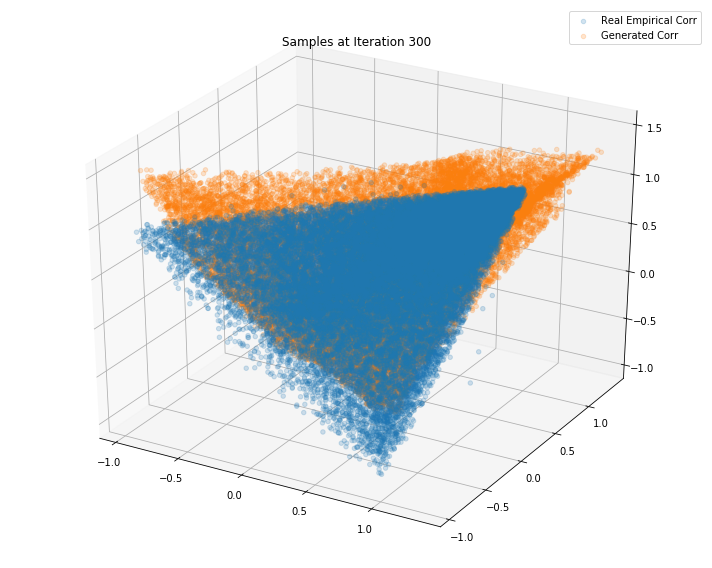``````Iterations: 400	 Discriminator loss: 1.3885	 Generator loss: 0.6952
````````````Iterations: 500	 Discriminator loss: 1.3843	 Generator loss: 0.7001
``````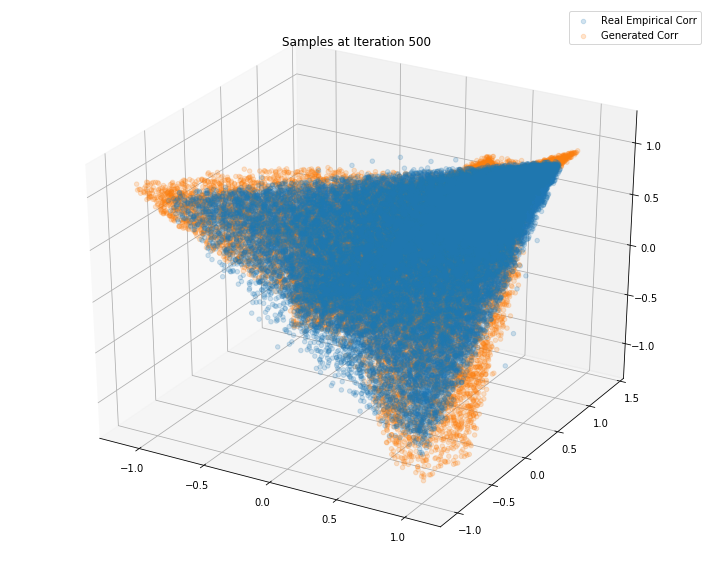``````Iterations: 600	 Discriminator loss: 1.3835	 Generator loss: 0.6997
````````````Iterations: 700	 Discriminator loss: 1.3832	 Generator loss: 0.7047
``````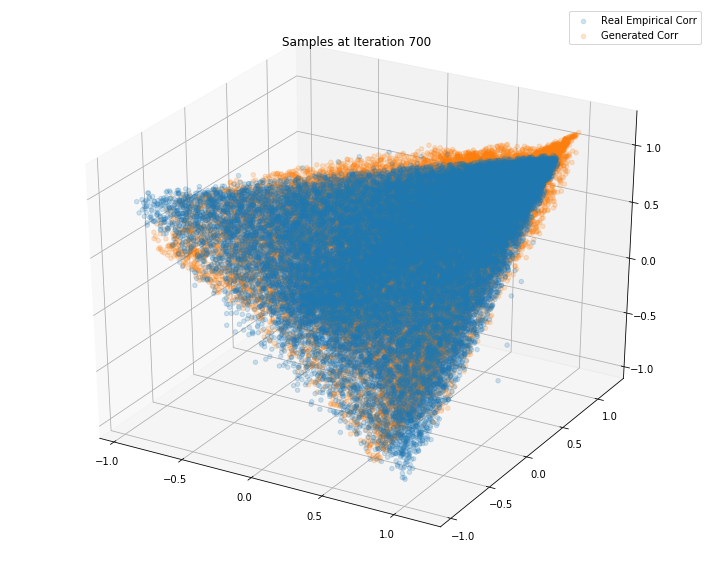``````Iterations: 800	 Discriminator loss: 1.3851	 Generator loss: 0.6845
``````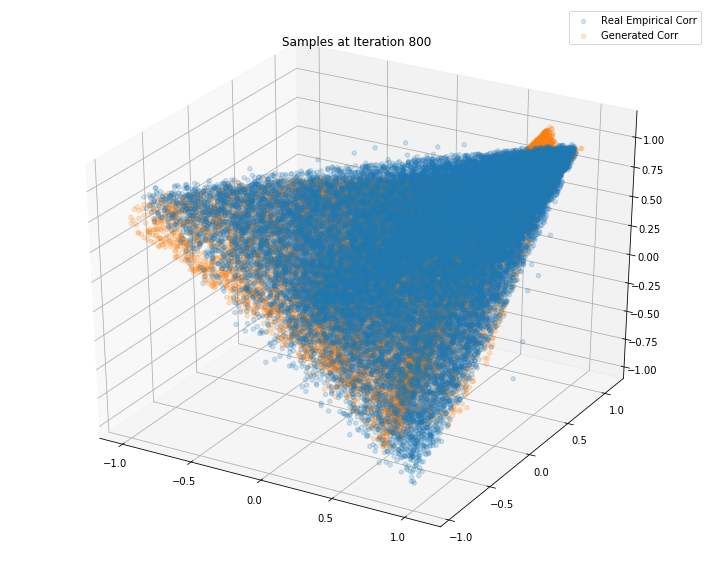``````Iterations: 900	 Discriminator loss: 1.3841	 Generator loss: 0.7035
````````````Iterations: 1000	 Discriminator loss: 1.3850	 Generator loss: 0.6881
````````````Iterations: 50000	 Discriminator loss: 1.3858	 Generator loss: 0.6898
````````````Iterations: 100000	 Discriminator loss: 1.3795	 Generator loss: 0.6682
``````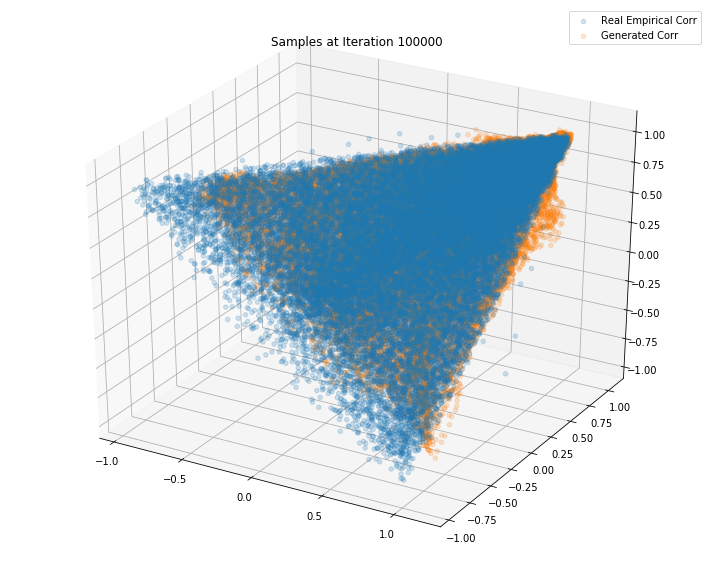``````Iterations: 150000	 Discriminator loss: 1.3700	 Generator loss: 0.6214
````````````Iterations: 200000	 Discriminator loss: 1.3853	 Generator loss: 0.7050
````````````n_dots = 2**12
x_plot = sample_corr(n=n_dots)
Z_plot = sample_Z(n_dots, 3)

fig = plt.figure(figsize=(10, 8))
g_plot = sess.run(G_sample, feed_dict={Z: Z_plot})